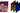Reading Notes - Ch. 4 - Fastbook (part 1)

There's one guaranteed way to fail, and that's to stop trying.

Images in Computers

Computers work with grayscale images as two-dimensional matrices. The dimensions of the matrix are the width and height of the image. Each value in the matrix is the intensity of that pixel between 0 (completely white) and 255 (completely black), or the other way (0 = completely black, 255 = completely white).

The maximum possible value is 255 because that's the biggest number that can be stored in 8 bits and each pixel in the image is represented using 8 bits (also known as 8-bit color depth). However, it is possible to also have each pixel represented using 10 bits (10-bit color depth), 12 bits (12-bit color depth), etc. In that case, the maximum value will be higher than 255.

Color images are most commonly stored using the RGB (Red, Green, Blue) color model. With this model, instead of a single two-dimensional array (like for grayscale images), an image is represented using three two-dimensional arrays - one for each of the colors. A color image is therefore represented as a three-dimensional matrix.Source: https://www.kdnuggets.com/2019/12/convert-rgb-image-grayscale.html

Baselines

A simple model that you are confident should perform reasonably well.

It should be very quick and easy to implement and test. Every improvement you make in your model afterwards can be compared against the performance of the baseline to ensure you are always doing better.

Two ways of building a baseline:

1. use a simple, easy-to-implement model
2. find code other people have written to solve a similar problem and run it with your data

Classifying Digits using Pixel Similarity

A simple, easy-to-implement model to use as a baseline for handwritten digit classification.

Use all images of a number in the training set to calculate the average value of each pixel in the number.

Then compare each pixel in the image you want to make a prediction for with the pixels of each of the "average images" of the digits and average all the values. The prediction will be the number whose "average image" is the least distance away.

When comparing each pixel value with an "average image" and then averaging the result, you can use the L1 norm or the RMSE. L1 applies the same penalty uniformly. RMSE penalizes larger errors more and smaller errors less.

Tensors

A tensor is a multidimensional array that has the same numeric type for all its components. In addition, it cannot be jagged (inner arrays having different sizes) - it always has a multi-dimensional rectangular shape.

Tensors have the capability of living on a GPU (if available) making computations much faster. They are also capable of automatically calculating derivatives of operations performed on them.

The rank of a tensor is how many dimensions it has.(Technically, the last image should be a rank-3 tensor. A scalar is a rank-0 tensor, a vector is a rank-1 tensor and a two-dimensional matrix is a rank-2 tensor)

The shape of a tensor shows us how many elements there are along each dimension.

When trying to perform an operation between two tensors of different ranks, PyTorch tries to use broadcasting.

1. Each tensor has at least one dimension.
2. When iterating over the dimension sizes, starting from the last dimension:
1. Both dimensions are equal
2. One of the dimensions is equal to 1
3. One of the dimensions doesn't exist (the tensor has a smaller rank)

If two tensors are "broadcastable", then the resulting tensor size will have a shape determined as follows:

• If the rank of the tensors are not equal, a dimension of 1 is prepended to the tensor with a smaller rank until the ranks are equal.
• For each dimensions, the size of the result is the maximum of the sizes of the two tensors along that dimension.